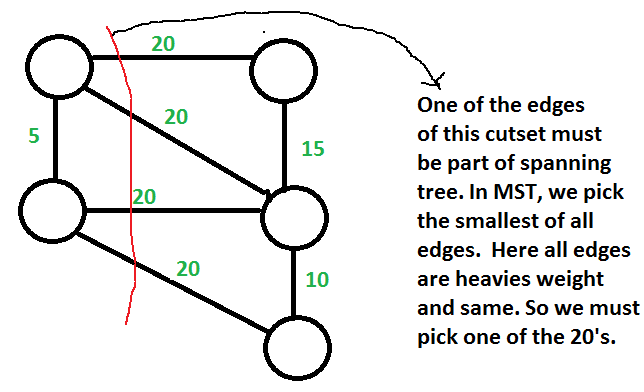Open In App

# GATE | Gate IT 2005 | Question 52

Let G be a weighted undirected graph and e be an edge with maximum weight in G. Suppose there is a minimum weight spanning tree in G containing the edge e. Which of the following statements is always TRUE?

(A) There exists a cutset in G having all edges of maximum weight.
(B) There exists a cycle in G having all edges of maximum weight
(C) Edge e cannot be contained in a cycle.
(D) All edges in G have the same weight

Explanation: Background : Given a connected and undirected graph, a spanning tree of that graph is a subgraph that is a tree and connects all the vertices together.

1. A spanning tree has exactly V – 1 edges.
2. A single graph can have many different spanning trees. A minimum spanning tree (MST) or minimum weight spanning tree for a weighted, connected and undirected graph is a spanning tree with weight less than or equal to the weight of every other spanning tree. The weight of a spanning tree is the sum of weights given to each edge of the spanning tree.
3. There can be more that one possible spanning trees of a graph. For example, the graph in this question has 6 possible spanning trees.
4. MST has lightest edge of every cutset. Remember Prim’s algorithm which basically picks the lightest edge from every cutset.

Choices of this question :
a) There exists a cutset in G having all edges of maximum weight : This is correct. If there is a heaviest edge in MST, then there exist a cut with all edges with weight equal to heavies edge. See point 4 discussed in above background.b) There exists a cycle in G having all edges of maximum weight : Not always TRUE. The cutset of heaviest edge may contain only one edge. In fact there may be overall one edge of heavies weight which is part of MST (Consider a graph with two components which are connected by only one edge and this edge is the heavies)

c) Edge e cannot be contained in a cycle. Not Always True. The curset may form cycle with other edges.

d) All edges in G have the same weight Not always True. As discussed in option b, there can be only one edge of heaviest weight.

Quiz of this Question